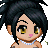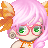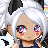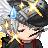• Send Message

## dapeacefulmanRegistered: 08/07/2008

Gender: Male

Birthday: 11/19/1991

## Friends

View All Friends

••••••••••## Scrubs: Steve Porter REMIX!

Report | 10/26/2013 9:46 amI hope you are well my old friend. been quit a few years but figured i would pop in here adn say hi lol.
Report | 05/16/2011 7:42 pmPfft, just like you to get on once and then never to return! xD

Hooppppee your having fun with whatever. (:
Report | 05/03/2011 8:28 pm```I missed you! And when i come on you get off? What has the world come to? XD

T_TT_TT_TT_TT_TT_TT_TT_TT_TT_TT_TT_TT_TT_TT_TT_TT_TT_TT_TT_TT_TT_TT_TT_TT_TT_TT_TT_TT_TT_TT_TT_TT_TT_TT_TT_TT_TT_TT_TT_TT_TT_TT_TT_TT_TT_TT_TT_TT_TT_TT_TT_TT_TT_TT_TT_TT_TT_TT_TT_TT_TT_TT_TT_TT_TT_TT_TT_TT_TT_TT_TT_TT_TT_TT_TT_TT_TT_TT_TT_TT_TT_TT_TT_TT_TT_TT_TT_TT_TT_TT_TT_TT_TT_TT_TT_TT_TT_TT_TT_TT_TT_TT_TT_TT_TT_TT_TT_TT_TT_TT_TT_TT_TT_TT_TT_TT_TT_TT_TT_TT_TT_TT_TT_TT_TT_TT_TT_TT_TT_TT_TT_TT_TT_TT_TT_TT_TT_TT_TT_TT_TT_TT_TT_TT_TT_TT_TT_TT_TT_TT_TT_TT_TT_TT_TT_TT_TT_TT_TT_TT_TT_TT_TT_TT_TT_TT_TT_TT_TT_TT_TT_TT_TT_TT_TT_TT_TT_TT_TT_TT_TT_TT_TT_TT_TT_TT_TT_TT_TT_TT_TT_TT_TT_TT_TT_TT_TT_TT_TT_TT_TT_TT_TT_TT_TT_TT_TT_TT_TT_TT_TT_TT_TT_TT_TT_TT_TT_TT_TT_TT_TT_TT_TT_TT_TT_TT_TT_TT_TT_TT_TT_TT_TT_TT_TT_TT_TT_TT_TT_TT_TT_TT_TT_TT_TT_TT_TT_TT_TT_TT_TT_TT_TT_TT_TT_TT_TT_TT_TT_TT_TT_TT_TT_TT_TT_TT_TT_TT_TT_TT_TT_TT_TT_TT_TT_TT_TT_TT_TT_TT_TT_TT_TT_TT_TT_TT_TT_TT_TT_TT_TT_TT_TT_TT_TT_TT_TT_TT_TT_TT_TT_TT_TT_TT_TT_TT_TT_TT_TT_TT_TT_TT_TT_TT_TT_TT_TT_TT_TT_TT_TT_TT_TT_TT_TT_TT_TT_TT_TT_TT_TT_TT_TT_TT_TT_TT_TT_TT_TT_TT_TT_TT_TT_TT_TT_TT_TT_TT_TT_TT_TT_TT_TT_TT_TT_TT_TT_TT_TT_TT_TT_TT_TT_TT_TT_TT_TT_TT_TT_TT_TT_TT_TT_TT_TT_TT_TT_TT_TT_TT_TT_TT_TT_TT_TT_TT_TT_TT_TT_TT_TT_TT_TT_TT_TT_TT_TT_TT_TT_TT_TT_TT_TT_TT_TT_TT_TT_TT_TT_TT_TT_TT_TT_TT_TT_TT_TT_TT_TT_TT_TT_TT_TT_TT_TT_TT_TT_TT_TT_TT_TT_TT_TT_TT_TT_TT_TT_TT_TT_TT_TT_TT_TT_TT_TT_TT_TT_TT_TT_TT_TT_TT_TT_TT_TT_TT_TT_TT_TT_TT_TT_TT_TT_TT_TT_TT_TT_TT_TT_TT_TT_TT_TT_TT_TT_TT_TT_TT_TT_TT_TT_TT_TT_TT_TT_TT_TT_TT_TT_TT_TT_TT_TT_TT_TT_TT_TT_TT_TT_TT_TT_TT_TT_TT_TT_TT_TT_TT_TT_TT_TT_TT_TT_TT_TT_TT_TT_TT_TT_TT_TT_TT_TT_TT_TT_TT_TT_TT_TT_TT_TT_TT_TT_TT_TT_TT_TT_TT_TT_TT_TT_TT_TT_TT_TT_TT_TT_TT_TT_TT_TT_TT_TT_TT_TT_TT_TT_TT_TT_TT_TT_TT_TT_TT_TT_TT_TT_TT_TT_TT_TT_TT_TT_TT_TT_TT_TT_TT_TT_TT_TT_TT_TT_TT_TT_TT_TT_TT_TT_TT_TT_TT_TT_TT_TT_TT_TT_TT_TT_TT_TT_TT_TT_TT_TT_TT_TTT_TT_TT_TT_TT_TT_TT_TT_TT_TT_TT_TT_TT_TT_TT_TT_TT_TT_TT_TT_TT_TT_TT_TT_TT_TT_TT_TT_TT_TT_TT_TT_TT_TT_TT_TT_TT_TT_TT_TT_TT_TT_TT_TT_TT_TT_TT_TT_TT_TT_TT_TT_TT_TT_TT_TT_TT_TT_TT_TT_TT_TT_TT_TT_TT_TT_TT_TT_TT_TT_TT_TT_TT_TT_TT_TT_TT_TT_TT_TT_TT_TT_TT_TT_TT_TT_TT_TT_TT_TT_TT_TT_TT_TT_TT_TT_TT_TT_TT_TT_TT_TT_TT_TT_TT_TT_TT_TT_TT_TT_TT_TT_TT_TT_TT_TT_TT_TT_TT_TT_TT_TT_TT_TT_TT_TT_TT_TT_TT_TT_TT_TT_TT_TT_TT_TT_TT_TT_TT_TT_TT_TT_TT_TT_TT_TT_TT_TT_TT_TT_TT_TT_TT_TT_TT_TT_TT_TT_TT_TT_TT_TT_TT_TT_TT_TT_TT_TT_TT_TT_TT_TT_TT_TT_TT_TT_TT_TT_TT_TT_TT_TT_TT_TT_TT_TT_TT_TT_TT_TT_TT_TT_TT_TT_TT_TT_TT_TT_TT_TT_TT_TT_TT_TT_TT_TT_TT_TT_TT_TT_TT_TT_TT_TT_TT_TT_TT_TT_TT_TT_TT_TT_TT_TT_TT_TT_TT_TT_TT_TT_TT_TT_TT_TT_TT_TT_TT_TT_TT_TT_TT_TT_TT_TT_TT_TT_TT_TT_TT_TT_TT_TT_TT_TT_TT_TT_TT_TT_TT_TT_TT_TT_TT_TT_TT_TT_TT_TT_TT_TT_TT_TT_TT_TT_TT_TT_TT_TT_TT_TT_TT_TT_TT_TT_TT_TT_TT_TT_TT_TT_TT_TT_TT_TT_TT_TT_TT_TT_TT_TT_TT_TT_TT_TT_TT_TT_TT_TT_TT_TT_TT_TT_TT_TT_TT_TT_TT_TT_TT_TT_TT_TT_TT_TT_TT_TT_TT_TT_TT_TT_TT_TT_TT_TT_TT_TT_TT_TT_TT_TT_TT_TT_TT_TT_TT_TT_TT_TT_TT_TT_TT_TT_TT_TT_TT_TT_TT_TT_TT_TT_TT_TT_TT_TT_TT_TT_TT_TT_TT_TT_TT_TT_TT_TT_TT_TT_TT_TT_TT_TT_TT_TT_TT_TT_TT_TT_TT_TT_TT_TT_TT_TT_TT_TT_TT_TT_TT_TT_TT_TT_TT_TT_TT_TT_TT_TT_TT_TT_TT_TT_TT_TT_TT_TT_TT_TT_TT_TT_TT_TT_TT_TT_TT_TT_TT_TT_TT_TT_TT_TT_TT_TT_TT_TT_TT_TT_TT_TT_TT_TT_TT_TT_TT_TT_TT_TT_TT_TT_TT_TT_TT_TT_TT_TT_TT_TT_TT_TT_TT_TT_TT_TT_TT_TT_TT_TT_TT_TT_TT_TT_TT_TT_TT_TT_TT_TT_TT_TT_TT_TT_TT_TT_TT_TT_TT_TT_TT_TT_TT_TT_TT_TT_TT_TT_TT_TT_TT_TT_TT_TT_TT_TT_TT_TT_TT_TT_TT_TT_TT_TT_TT_TT_TT_TT_TT_TT_TT_TT_TT_TT_TT_TT_TT_TT_TT_TT_TT_TT_TT_TT_TT_TT_TT_TT_TT_TT_TT_TT_TT_TT_TT_TT_TT_TT_TT_TT_TT_TT_TT_TT_TT_TT_TT_TT_TT_TT_TT_TT_TT_TT_TT_TT_TT_TT_TT_TT_TT_TT_TT_TT_TT_TT_TT_TT_TT_TT_TT_TT_TT_TT_TT_TT_TT_TT_TT_TT_TT_TT_TT_TT_TT_TT_TT_TT_TT_TT_TT_TT_TT_TT_TT_TT_TT_TT_TT_TT_TT_TT_TT_TT_TT_TT_TT_TT_TT_TT_TT_TT_TT_TT_TT_TT_TT_TT_TT_TT_TT_TT_TT_TT_TT_TT_TT_TT_TT_TT_TT_TT_TT_TT_TT_TT_TT_TT_TT_TT_TT_TT_TT_TT_TT_TT_TT_TT_TT_TT_TT_TT_TT_TT_TT_TT_TT_TT_TT_TT_TT_TT_TT_TT_TT_TT_TT_TT_TT_TT_TT_TT_TT_TT_TT_TT_TT_TT_TT_TT_TT_TT_TT_TT_TT_TT_TT_TT_TT_TT_TT_TT_TT_```
Report | 05/01/2011 7:08 pmAwwww i know!! D;

I HAVE MISSED YOU SOOOOOOOOOO MUCH!! <3

BUT I AM SORRY TO SAY I WILL NOT BE COMING ON AS MUCH. T~T
Report | 02/03/2011 5:13 pmAndy... D:<

Hahaha you come on.. and leave.. forever. Dx
Report | 01/01/2011 9:03 pmit was good to hear from you. I hope maybe on a diff site since ur not on here much we can keep in contact. have a great day bud smile
Report | 12/17/2010 2:15 pmOh hey Andy! :3
Report | 11/03/2010 7:17 pmits been a long time Andy. i looked on here and realized we were both gone a long time. it stll said i was 22 on my profile lol. we both changed and moved on in life and age I imagine. anyways if u ever get on and see this. know u will always be my forever friend smile mrgreen
Report | 10/24/2010 10:35 amHey Mr. I Never Have Time To Get On Anymore. ;P

Report | 10/17/2010 6:33 pmHey Andy. :]